# Two-scale numerical simulation of a large deforming fluid-saturated porous structure¶

## Mathematical model¶

We consider a fluid-saturated porous medium undergoing large deformations. A double porous medium is constituted by a hyperelastic skeleton and an incompressible viscous fluid. Within the Eulerian framework related to the current deformed configuration, the two-scale homogenization approach is applied to a linearized model discretized in time, being associated with an incremental formulation. The homogenization procedure of the linearized equations provides effective (homogenized) material properties which are computed to constitute the incremental macroscopic problem. The multiscale model is derived and discussed in [LukesRohan2022].

## Implementation¶

The local subproblems defined within the reference cell and the global macroscopic equations are discretized and solved by means of the finite element method. The macroscopic equations and the incremental ULF algorithm are defined in `largedef_porous_mac.py`. The finite element mesh representing the macroscopic domain and the applied boundary conditions are depicted in Fig. 1.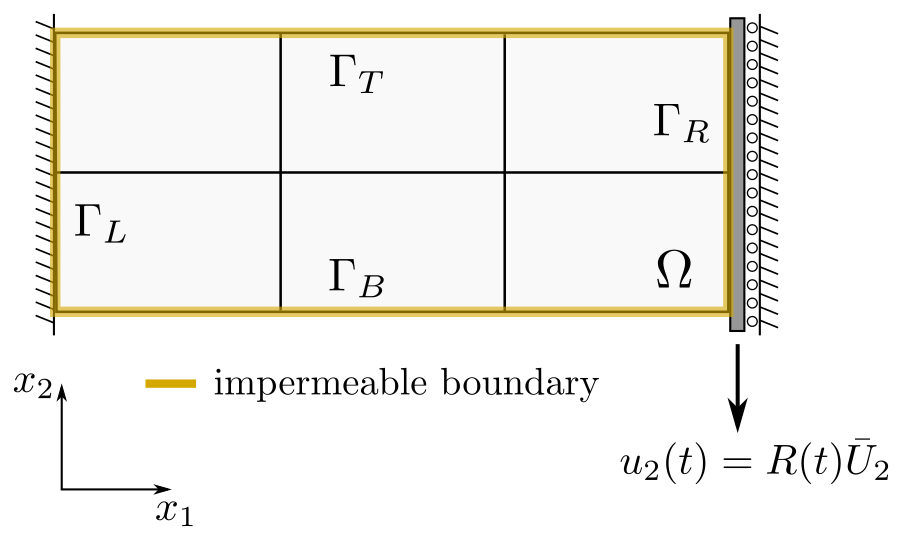Fig. 1 Boundary conditions applied to the macroscopic 2D sample.

The local microscopic problems and the homogenized coefficients are specified in `largedef_porous_mic.py`. The domain, on which the microscopic calculations are performed, is shown in Fig. 2. It consists of the porous matrix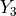and two fluid channels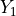,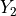.

The macro-micro coupling is governed by the SfePy homogenization engine, see [CimrmanLukesRohan2019], which ensures efficient evaluation of the homogenized coefficients demanded by the macroscopic simulation.Fig. 2 Decomposition of the microscopic domain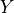.

## Running simulation¶

To run the multiscale numerical simulation, download the archive, unpack it in the main SfePy directory and type:

```./simple.py example_largedef_porous-1/largedef_porous_mac.py
```

This invokes the time-stepping solver for the problem at the macroscopic level which calls the homogenization engine evaluating the homogenized coefficients defined in `largedef_porous_mic.py`. The coefficients are evaluated in several embedded loops associated with the time steps and the macroscopic integration points, see [LukesRohan2022] for details.

The computed macroscopic results can be visualized using the `resview.py` post-processing script as follows:

```./resview.py example_largedef_porous-1/results/macro_mesh_3x2_*.vtk -w u0 -f cauchy_stress:p0 -v "0,0" -s 18
```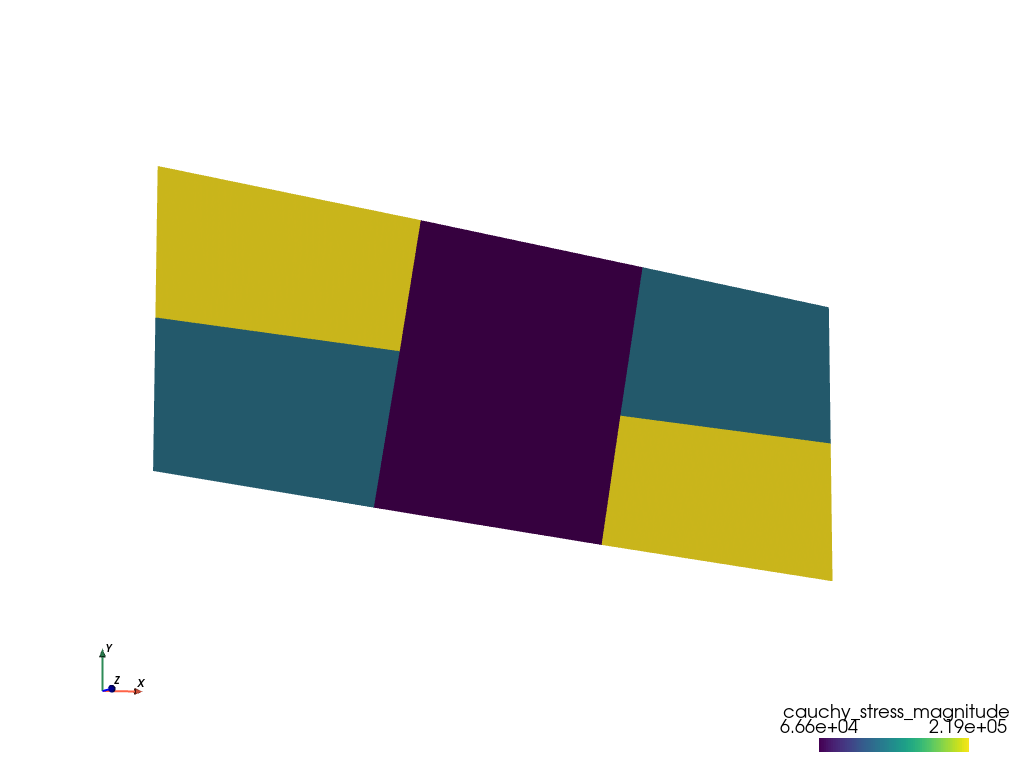Fig. 3 Distribution of the Cauchy stress magnitude (average values per element) in the macroscopic deformed domain at time step 18.